Thu, Sep 29, 2022 @ 07:40 GMT
HomeContributorsTechnical AnalysisTechnical Outlook and Review

# Technical Outlook and Review

USD/JPY:

On the H4 chart, price has confirmed a bullish momentum breaking the previous high. We are bullish bias- Price has tested the first resistance at 138.870 where the previous swing high and 161.8% extension sits. If bullish momentum continues, it will bring price to 139.390 where the previous swing high sits. Alternatively, if price reverse, it might pull back to test the first support at 136.490 where the 23.6% fibonacci retracement sits then subsequently the second support at 135.398 where the 38.2% retracement sits

Areas of consideration:

• H4 time frame, 1st resistance at 138.870
• H4 time frame, 1st support at 136.490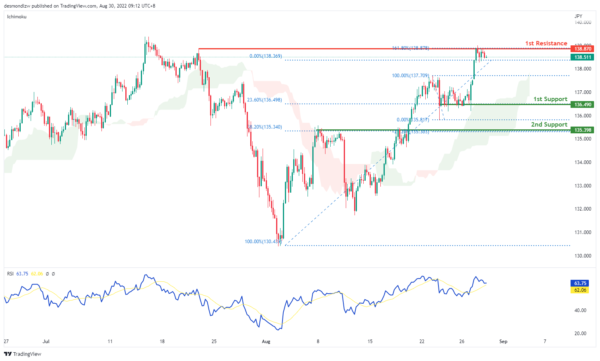DXY:

On the H4, price is still moving in an ascending trend and is in a bullish momentum. Price has tested the first resistance at 109.440 where the 78.6% Fibonacci projection and the previous swing high sits. If price fails to break this level, it will pull back to test the first support at 107.273 where the 50% retracement and 100% projection sits and subsequently the second support at 106.235 where the 61.8% retracement sits

Areas of consideration:

• H4 time frame, 1st resistance at 109.440
• H4 time frame, 1st support at 107.273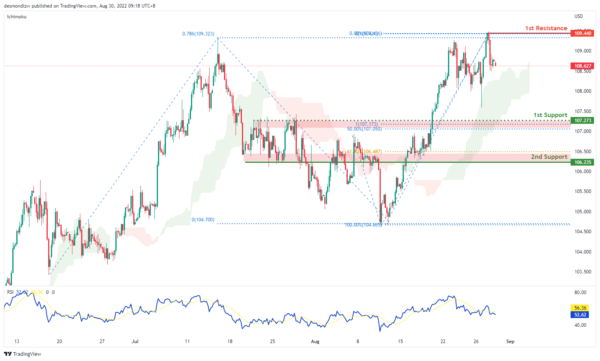EUR/USD:

On the H4, prices have tested the first support again at 0.9904 where the 61.8% projection sits. Prices seem to have formed a triple bottom and have reversed – we are in a slightly bullish momentum. Price look like it’s pulling back to test the first resistance at 1.0126 where the previous swing low and 50% retracement sits and subsequently the second resistance at 1.0258 where the 78.6% retracement and swing high sits

Areas of consideration :

• H4 1st resistance at 1.0126
• H4 1st support at 0.9904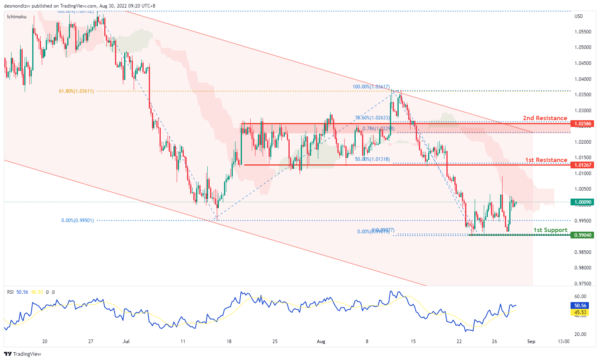GBP/USD:

On the H4, prices seem to be in a bearish momentum and respecting the ichimoku cloud. Prices have broken the 78.6% projection level and have tested the first support, 100% projection at 1.1632 levels. Price has pulled back slightly and might look to test the first resistance at 1.1854 level where the 38.2% retracement sit then the second resistance at 1.1933 level where the previous swing low and 127.2% extension sits

Areas of consideration:

• H4 1st resistance at 1.1854
• H4 1st support at 1.1632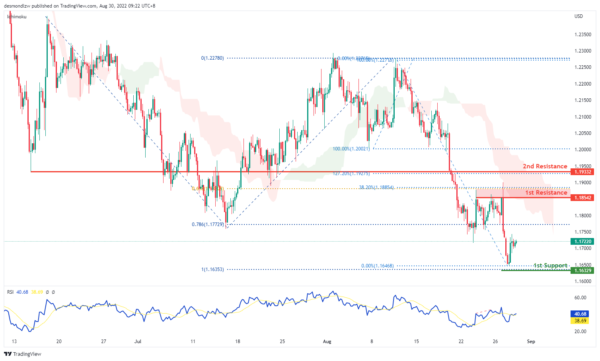USD/CHF:

On the H4, with prices moving above the ichimoku cloud and breaking the descending trend, we are bullish bias. We’re looking at price to test the first resistance at 0.9734 where the 127.2% extension sits. If price continues with bullish momentum, it will bring price to second resistance at 0.9852. Alternatively, prices could pull back to test the first support at 0.96282 where the 23.6% fibonacci retracement and previous swing low sits then the second support at 0.9491 where the 61.8% retracement and 78.6% projection sits

Areas of consideration

• H4 1st support at 0.9628
• H4 1st resistance at 0.9734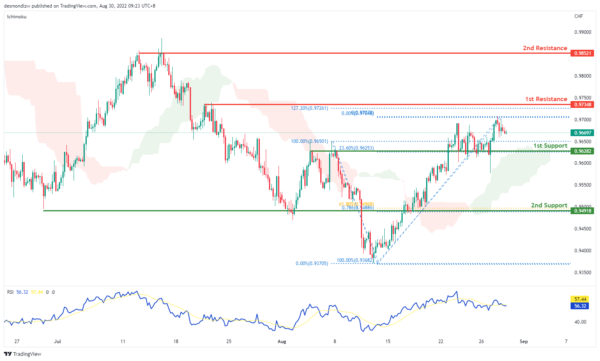XAU/USD (GOLD):

On the H4, with prices moving below ichimoku cloud and long term descending trendline, we have a bearish bias. As the price is above MA and MACD is showing a golden cross signal, we can expect the price bullback to the 1st resistance at 1743.892 first, which is in line with the 61.8% fibonacci projection and then drop to the 1st support at 1722.026, where the swing low is. If the price breaks the 1st support, we can expect the price drop to the 2nd support at 1707.850, where the 78.6% fibonacci retracement is. Alternatively, the price may break the 1st resistance at 1743.892 and then rise to the 2nd resistance at 1764.955, where the swing high is.

Areas of consideration:

• H4 time frame, 1st resistance at 1743.892
• H4 time frame, 1st support at 1722.026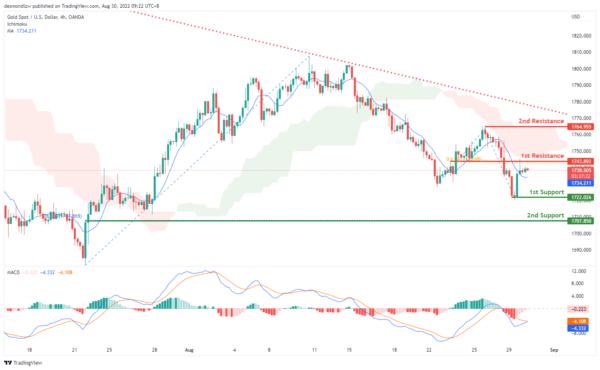AUD/USD:

On the H4, with the price moving below the ichimoku cloud and moving within the descending channel, we have a bearish bias that the price may drop from the 1st support at 0.68729, which is in line with the swing lows and 61.8% fibonacci retracement to the 2nd support at 0.67916, where the overlap support and 78.6% fibonacci projection are. Alternatively, the price may rise to the 1st resistance at 0.69411, where the 61.8% fibonacci projection and previous swing high are. If the price can break this resistance level, we can expect the price to rise to the 2nd resistance at 0.69925, where the swing highs, 50% fibonacci retracement and 100% fibonacci projection are.

Areas of consideration

• H4 1st support at 0.68729
• H4 2nd support at 0.67916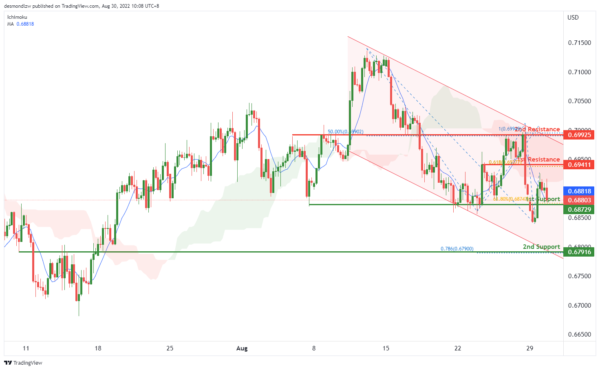NZD/USD:

On the H4, with price moving within the descending trendline, below the ichimoku indicator and breaking the MA, we have a bearish bias that price may drop to 1st support at 0.61026, where the swing low is to the 2nd support at 0.60649, where the 78.6% fibonacci projection is. Alternatively, price could rise to 1st resistance at 0.61923, which is in line with 61.8% fibonacci retracement.

Areas of consideration:

• H4 time frame, 1st support at 0.61026
• H4 time frame, 2nd support at 0.60649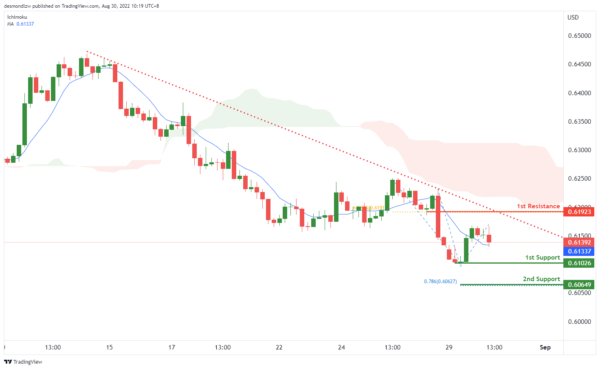On the H4, with the price moving within the ascending channel, above ichimoku cloud, we have a bullish bias that the price may rise from the 1st support at 1.29944, where the 23.6% fibonacci retracement and swing low are to the 1st resistance at 1.30812, where the swing highs are. If the price can break the 1st resistance, we can expect the momentum to carry the price to the 2nd resistance at 1.31657, where the swing high and 100% fibonacci projection are. Alternatively, price could break the 1st support and drop to the 2nd support at 1.29006, where the 50% fibonacci retracement and 100% fibonacci projection are.

Areas of consideration:

• H4 time frame, 1st support at 1.29944
• H4 time frame, 1st resistance at 1.30812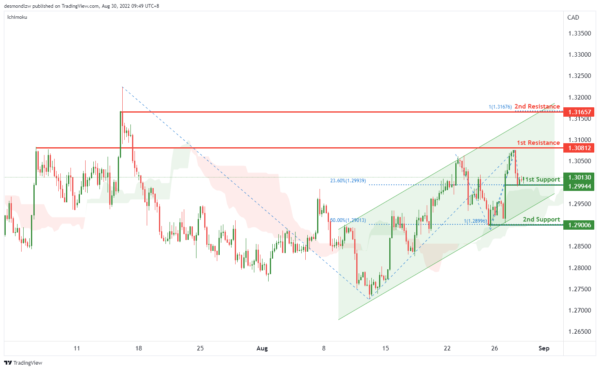OIL:

On the H4, with price moving within the ascending trendline, above ichimoku cloud and MA, we have a bullish bias that the price may rise from the 1st support at 102.989, which is in line with the overlap support to the 1st resistance at 105.923, where the swing highs and 100% fibonacci projection are. If the price breaks the 1st resistance, we can expect the price to rise to the 2nd resistance at 109.187, where the previous swing highs are. Alternatively, the price may drop to the 2nd support at 100.415, which is in line with the 38.2% fibonacci retracement.

Areas of consideration:

• H4 time frame, 1st support at 102.989
• H4 time frame, 1st resistance at 105.923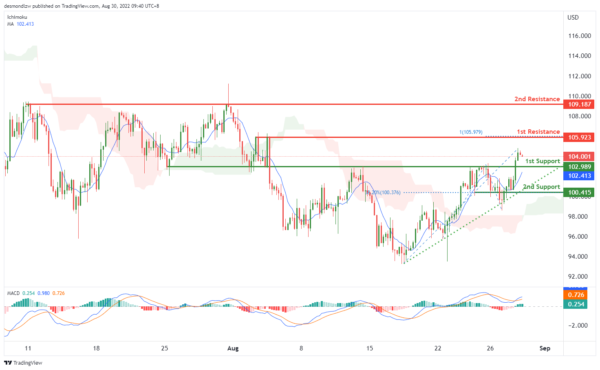Dow Jones Industrial Average:

On the H4, with price breaking the ascending trendline and moving below the ichimoku indicator, ,we have a bearish bias that price will drop to 1st support at 31904 where the pullback support, 100% fibonacci projection, 61.8% fibonacci retracement and 127.2% fibonacci extension are. Should price break 1st support, we would expect bearish momentum to carry price to 2nd support at 30467 where the pullback support is. Alternatively, price could rise to 1st resistance at 32623 in line with pullback resistance.

Areas of consideration:

• H4 time frame, 1st resistance of 32623
• H4 time frame, 1st support at 3190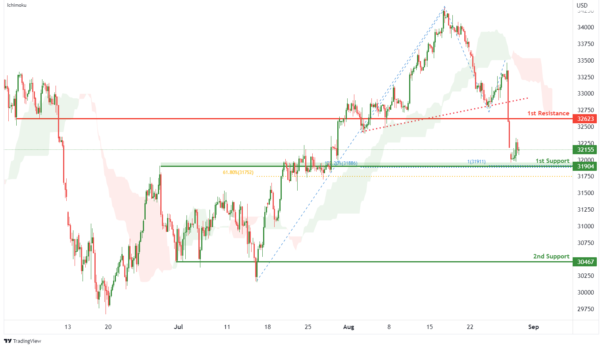DAX:

On the H4, with price moving within a descending channel and below the ichimoku indicator, we have a bearish bias that price will drop from 1st resistance at 13025.67 where the pullback resistance, 23.6% fibonacci retracement and 61.8% fibonacci projection are to the 1st support at 12399.72 where the swing low support and 161.8% fibonacci extension are. Alternatively, price could rise to 2nd resistance at 13378.95 where the overlap resistance and 50% fibonacci retracement are.

Areas of consideration:

• H4 time frame, 1st resistance of 13025.67
• H4 time frame, 1st support at 12399.72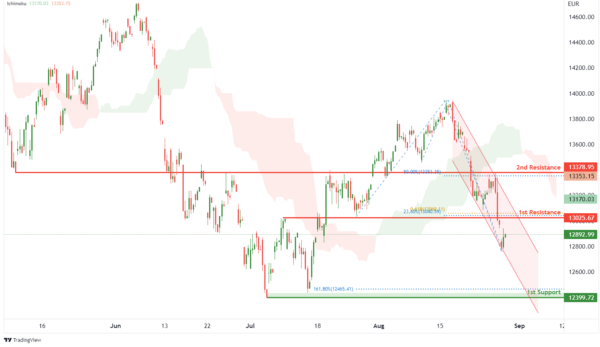ETHUSD:

On the H4, with price moving below the ichimoku indicator, we have a bearish bias that price will drop from 1st resistance at 1559.82 where the overlap resistance, 50% fibonacci retracement and 78.6% fibonacci projection are to the 1st support at 1419.94 where the swing low support, 161.8% fibonacci extension and 61.8% fibonacci projection are. Alternatively, price could break 1st resistance structure and rise to 2nd resistance at 1655.51 where the pullback resistance and 78.6% fibonacci retracement are.

Areas of consideration:

• H4 time frame, 1st resistance of 1559.82
• H4 time frame, 1st support at 1419.94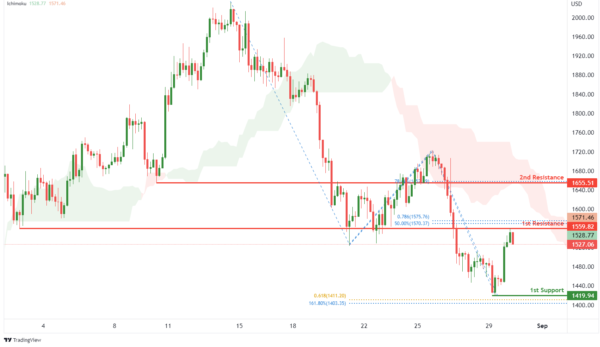BTCUSD:

On the H4, with price moving below an ichimoku indicator, we have a bearish bias that price will drop from 1st resistance at 20708.23 where the pullback resistance and 23.6% fibonacci retracement are to the 1st support at 18865.89 where the swing low support and -61.8% fibonacci expansion are. Take note of intermediate support at 19498.02 where the 127.2% fibonacci extension and swing low support are. Alternatively, price could break 1st resistance structure and rise to 2nd resistance at 22363.07 where the overlap resistance, 100% fibonacci projection and 50% fibonacci retracement are.

Areas of consideration:

• H4 time frame, 1st resistance of 20708.23
• H4 time frame, 1st support at 18865.89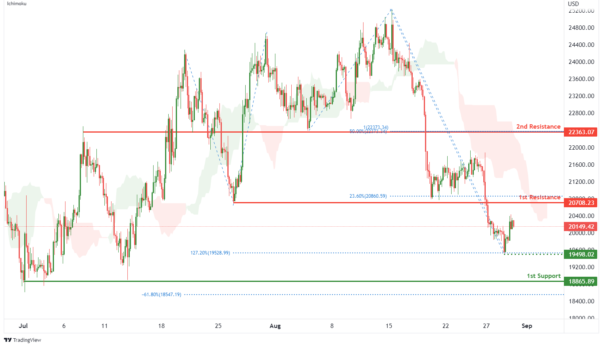S&P 500:

On the H4, with prices breaking out of the ascending trendline, we have a bearish bias that price will drop from 1st resistance at 4089.97 where the pullback resistance is to the 1st support at 3945.01 where the pullback support and 61.8% fibonacci retracement are. Alternatively, price could rise to 2nd resistance at 4182.68 where the pullback resistance is.

Areas of consideration:

• H4 time frame, 1st resistance of 4089.97
• H4 time frame, 1st support at 3945.01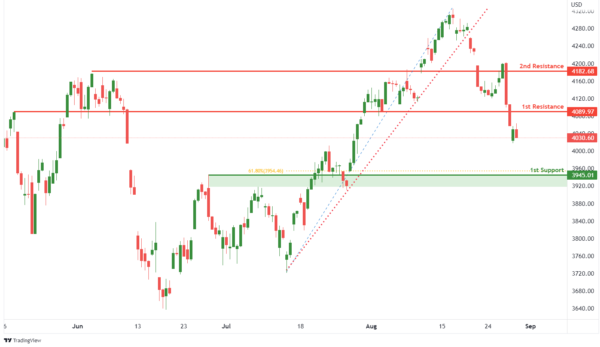IC Marketshttp://www.icmarkets.com/
IC Markets is revolutionizing on-line forex trading; on-line traders are now able to gain access to pricing and liquidity previously only available to investment banks and high net worth individuals.